Published

Tap & Drag Robotic Vacuum

A robotic vacuum made to help the elderly. We used Drawing Route for Car on the Office's Map (made by phpoc_man).

IntermediateWork in progress10 hours835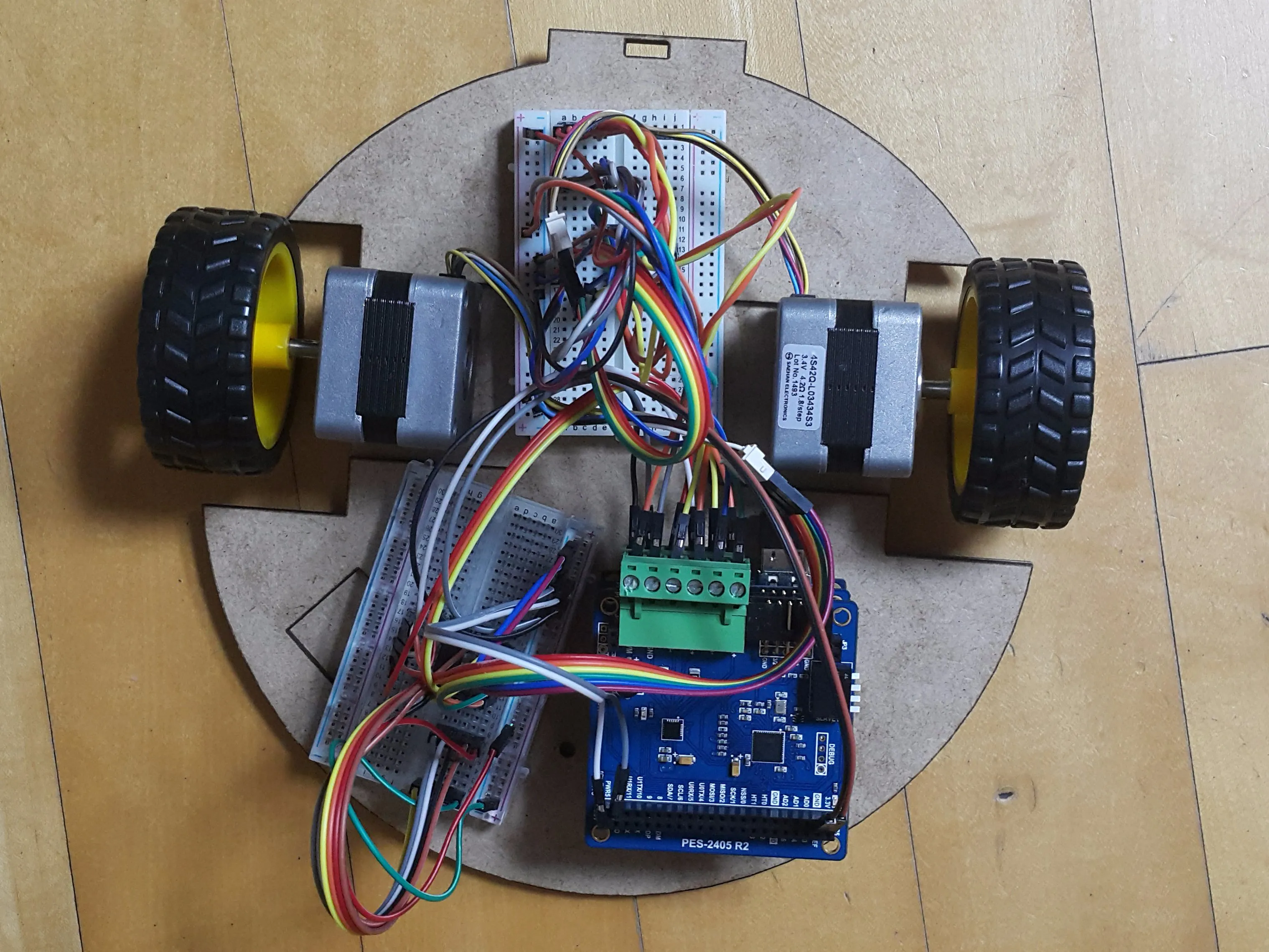Things used in this project

Hardware components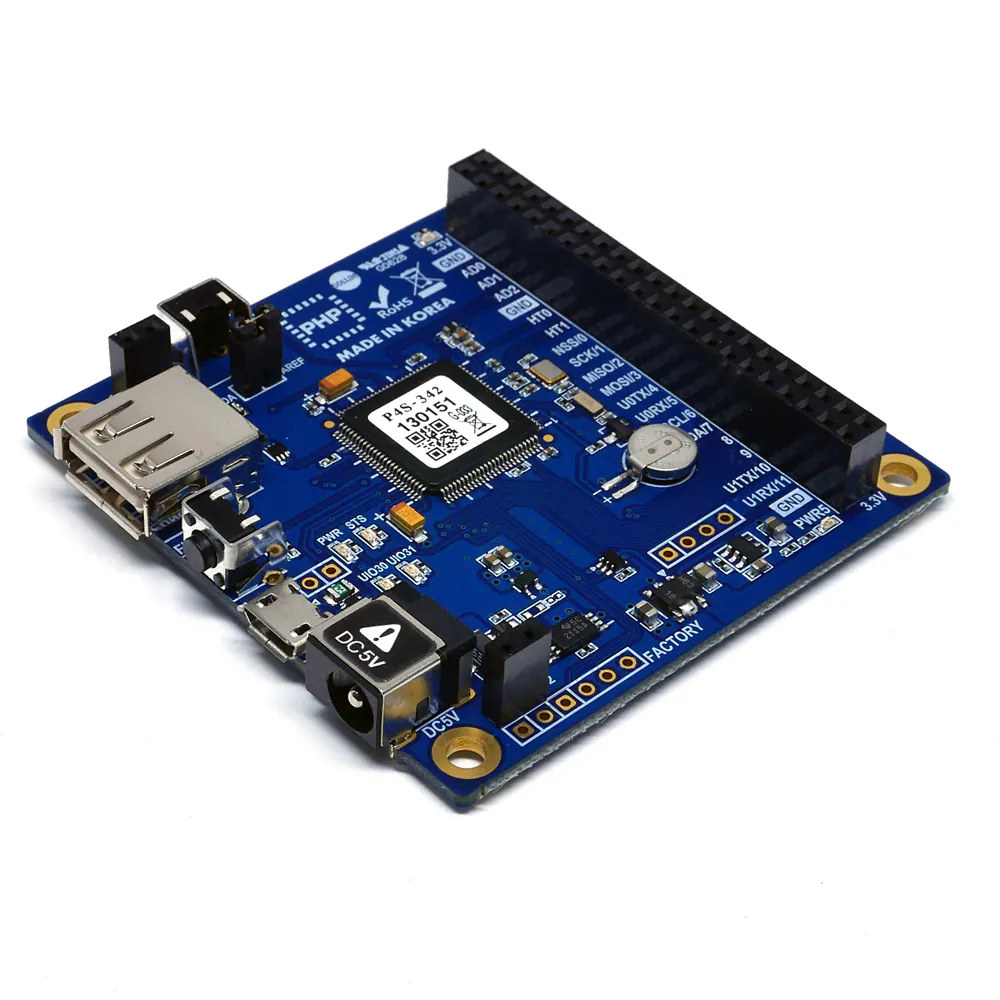PHPoC Blue
×1PHPoC Stepper Motor Controller Ⅱ (T-type)
×2
 step moter (4s42q-l03434s3)
×2Jumper wires (generic)
×1
 battery (used for phpoc and moter)
×2
 screw (or anything to attach your moters or boards to your car body. Like tape.)
×1

Software apps and online servicesPHPoC Debugger

Hand tools and fabrication machines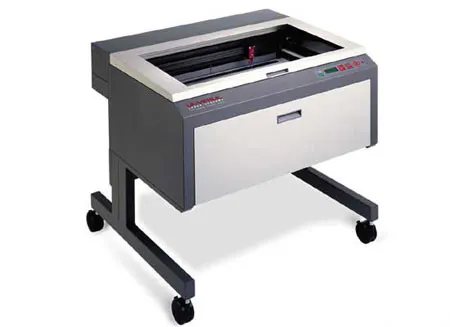Laser cutter (generic)

body

Schematics

20171029_213218_kNjCYJLl2X.jpg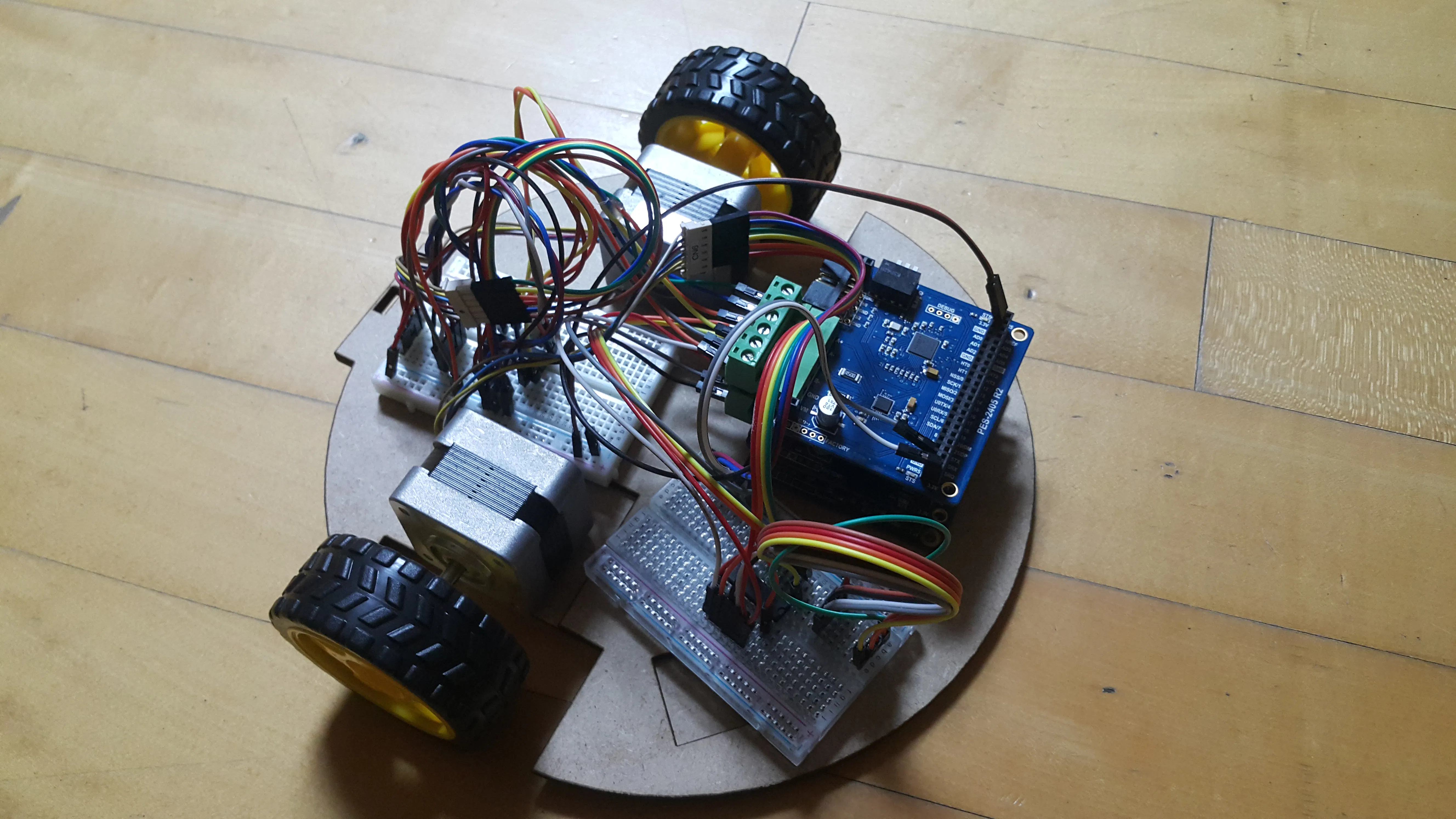20171029_213224_8W8votdH4Z.jpg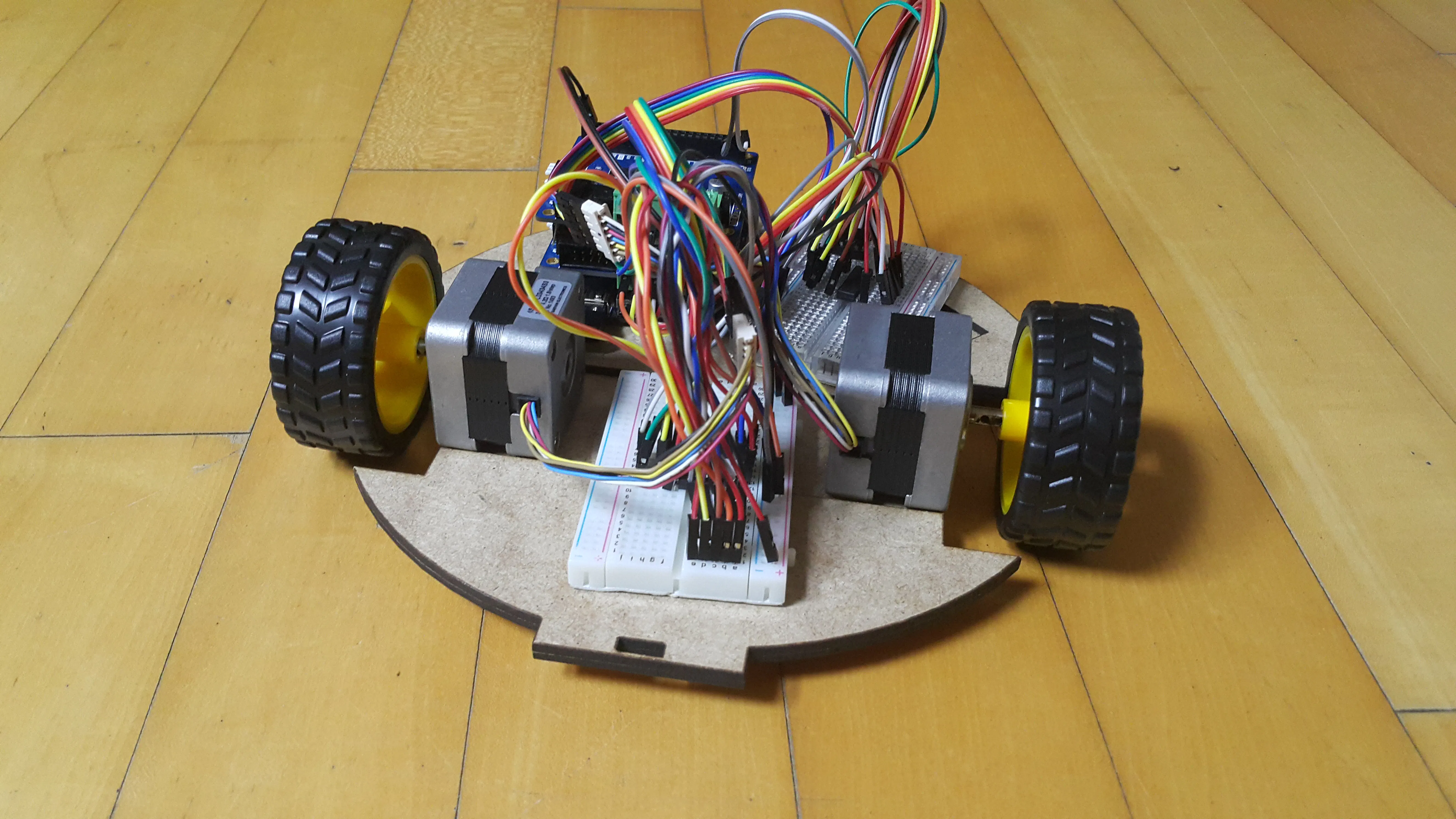20171029_213233_qIyP0BdmCl.jpg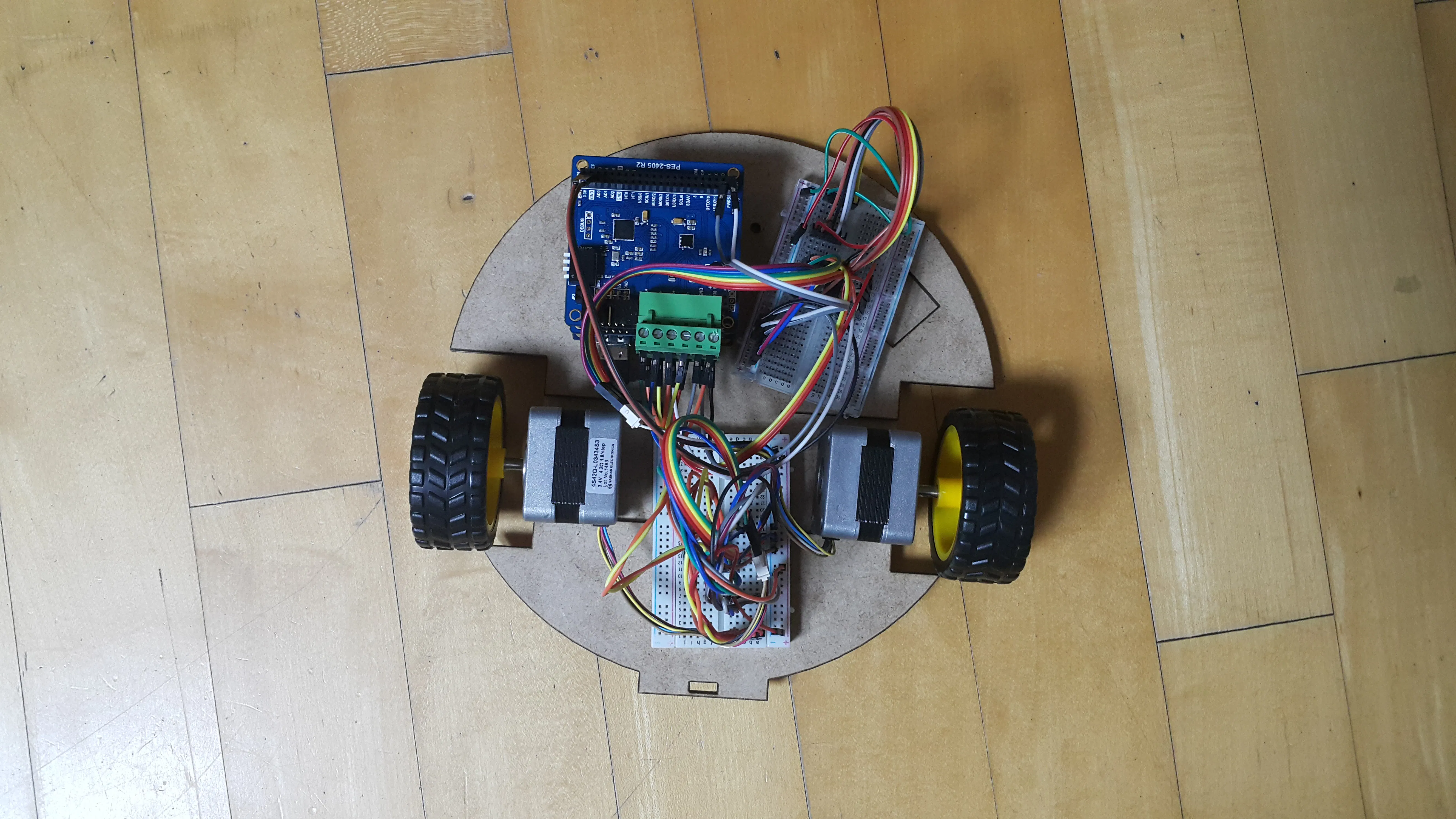Code

PHP
<?php
if(_SERVER("REQUEST_METHOD"))
exit; // avoid php execution via http request

include_once "/lib/sd_340.php";
include_once "/lib/sd_spc.php";
include_once "/lib/sn_tcp_ws.php";

define("TRACK",				300); //in mm
define("WHEEL_RADIUS",		65); // in mm
define("WHEEL_PERIMETER",	2*M_PI*WHEEL_RADIUS); // in mm

define("FULL_STEP_ANGLE",	1.8); //degree0
define("HALF_STEP_ANGLE",	0.9); //degree
define("FULL_STEP_NUM",		200); //step per round
define("HALF_STEP_NUM",		400); //step per round

define("MAP_IMG_WIDTH",		1140); //pixel
define("MAP_REAL_WIDTH",	25*450.0); //millimeter

define("STATE_FREE",		0);
define("STATE_PAUSE",		1);
define("STATE_MOVE",		2);

define("INF_DISTANCE",		1000); // 1000cm

function step_cmd(\$sid, \$cmd)
{
\$resp = spc_request(\$sid, 4, \$cmd);

if(\$resp)
{
\$resp = explode(",", \$resp);

if((int)\$resp != 200)
echo "step_cmd : '\$cmd' request error ", \$resp, "\r\n";

if(count(\$resp) > 1)
return \$resp;
else
return "";
}
else
return "";
}

function car_goto(\$target_x, \$target_y)
{
global \$pre_x, \$pre_y;
global \$pre_vector_x, \$pre_vector_y;
global \$target_step_1, \$target_step_2;
global \$car_state;

// calculate the rotate angle
\$vector_x  = \$target_x - \$pre_x;
\$vector_y  = \$target_y - \$pre_y;

\$length_1 = sqrt(\$pre_vector_x * \$pre_vector_x + \$pre_vector_y * \$pre_vector_y);
\$length_2 = sqrt(\$vector_x * \$vector_x + \$vector_y * \$vector_y);
\$cosin_alpha = (\$pre_vector_x * \$vector_x + \$pre_vector_y * \$vector_y) / ( \$length_1 * \$length_2);
\$angle = acos(\$cosin_alpha) * 180 / M_PI;

\$dir = \$pre_vector_x * \$vector_y - \$pre_vector_y * \$vector_x;

//save new values
\$pre_x = \$target_x;
\$pre_y = \$target_y;
\$pre_vector_x = \$vector_x;
\$pre_vector_y = \$vector_y;

\$step_num = (\$angle * TRACK) / (HALF_STEP_ANGLE * WHEEL_RADIUS) /2;

if(\$dir < 0)
{
\$target_step_1 += \$step_num;
\$target_step_2 += \$step_num;
}
else
{
\$target_step_1 -= \$step_num;
\$target_step_2 -= \$step_num;
}

\$pos_1 = round(\$target_step_1);
\$pos_2 = round(\$target_step_2);

step_cmd(13, "goto \$pos_1 400 1600");
step_cmd(14, "goto \$pos_2 400 1600");

while((int)step_cmd(13, "get state"))
usleep(1);
while((int)step_cmd(14, "get state"))
usleep(1);

\$dist = sqrt(\$vector_x*\$vector_x + \$vector_y*\$vector_y);

//millimeter to step
\$step_num = \$dist / WHEEL_PERIMETER * HALF_STEP_NUM;

\$target_step_1 += \$step_num;
\$target_step_2 -= \$step_num;

\$pos_1 = round(\$target_step_1);
\$pos_2 = round(\$target_step_2);

step_cmd(13, "goto \$pos_1 400 1600");
step_cmd(14, "goto \$pos_2 400 1600");

\$car_state = STATE_MOVE;
}

function sensor_setup()
{
// setup trigger pulse timer
ht_ioctl(0, "set mode output pulse");
ht_ioctl(0, "set div us");
ht_ioctl(0, "set repc 1");
ht_ioctl(0, "set count 5 10"); // 10us pulse width

// setup echo capture timer
ht_ioctl(1, "reset");
ht_ioctl(1, "set div us");
ht_ioctl(1, "set mode capture toggle");
ht_ioctl(1, "set trigger from pin rise");
ht_ioctl(1, "set repc 4");
}

{
ht_ioctl(1, "start"); // we should start capture timer first
ht_ioctl(0, "start"); // start trigger pulse

usleep(100000); // sleep 100ms
ht_ioctl(1, "stop");

// 1st capture value ("get count 0") is always zero.
// we should get 2nd capture value;
\$us = ht_ioctl(1, "get count 1");

if(\$us == 0)
\$dist = INF_DISTANCE;
else
{
\$dist = \$us * 340.0 / 2; // us to meter conversion
\$dist = \$dist / 10000; // meter to centimeter conversion
}

return \$dist;
}

function car_loop()
{
global \$target_step_1, \$target_step_2;
global \$car_state;

if(\$car_state == STATE_FREE)
return;

\$pos_1 = round(\$target_step_1);
\$pos_2 = round(\$target_step_2);

{
if(\$car_state == STATE_MOVE)
{
\$car_state = STATE_PAUSE;

step_cmd(13, "stop");
step_cmd(14, "stop");
}

return;
}
else if(\$car_state == STATE_PAUSE)
{
\$car_state = STATE_MOVE;

step_cmd(13, "goto \$pos_1 400 1600");
step_cmd(14, "goto \$pos_2 400 1600");
}

\$cur_step_1 = (float)step_cmd(13, "get pos");
\$cur_step_2 = (float)step_cmd(14, "get pos");

if(\$cur_step_1 == \$pos_1 && \$cur_step_2 == \$pos_2)
\$car_state = STATE_FREE;
}

function network_loop()
{
if(ws_state(0) == TCP_CONNECTED)
{
\$rbuf = "";
\$rlen = ws_read_line(0, \$rbuf);

if(\$rlen)
{
\$data = explode(" ", \$rbuf);
\$target_x = (int)\$data;
\$target_y = (int)\$data;

//pixel to millimeter
\$ratio = MAP_IMG_WIDTH / MAP_REAL_WIDTH; //pixel / millimeter
\$target_x /= \$ratio;
\$target_y /= \$ratio;

car_goto(\$target_x, \$target_y);
}
}
}

sensor_setup();
ws_setup(0, "step_motor_car", "csv.phpoc");
spc_reset();
spc_sync_baud(460800);

step_cmd(13, "set vref stop 2");
step_cmd(13, "set vref drive 14");
step_cmd(13, "set mode half");
step_cmd(13, "set rsnc 120 250");

step_cmd(14, "set vref stop 2");
step_cmd(14, "set vref drive 14");
step_cmd(14, "set mode half");
step_cmd(14, "set rsnc 120 250");

\$pre_x = 16*450.0;
\$pre_y = 22*450.0;
\$pre_vector_x = 0;
\$pre_vector_y = 1;

\$target_step_1 = 0.0;
\$target_step_2 = 0.0;

\$car_state = STATE_FREE;

while(1)
{
car_loop();

if(\$car_state == STATE_FREE)
network_loop();
}

?>

index.php

HTML
<!DOCTYPE html>
<html>
<title>PHPoC - Step Motor</title>
<meta name="viewport" content="width=device-width, initial-scale=0.7">
<style>
body {
text-align: center;
background-color: #33C7F2;
}
#cvs_frame {
margin-right: auto;
margin-left: auto;
position: relative;
background-color: #FFFFFF;
}
.canvas {
position: absolute;
left: 0px;
top: 0px;
overflow-y: auto;
overflow-x: hidden;
-webkit-overflow-scrolling: touch; /* nice webkit native scroll */
}

#layer_1 {
z-index: 2;
}
#layer_2 {
z-index: 1;
background: url(map.png) no-repeat;
background-size: contain;
border: 1px solid #000;
}

</style>
<script>
var CMD_MOVE = 2;
var RESOLUTION = 20;

var IMAGE_WIDTH = 1140, IMAGE_HEIGHT = 1562;

var ws = null;
var layer_1 = null, layer_2 = null;
var cvs_frame = null;
var ctx1 = null, ctx2 = null;

var canvas_width = 0, canvas_height = 0;

var x = 0, y = 0; //position in image coordinate (pixel)
var touch_x = 0; touch_y = 0; //position in canvas coordinate (in pixel).

var cvs_pos_x = 0, cvs_pos_y = 0;
var pre_cvs_pos_x = 0, pre_cvs_pos_y = 0;

function init()
{
//initial position
x = 16 * IMAGE_WIDTH / 25.0;
y = 22 * IMAGE_HEIGHT / 34.0;

cvs_frame = document.getElementById("cvs_frame");

layer_1 = document.getElementById("layer_1");
layer_2 = document.getElementById("layer_2");

ctx1 = layer_1.getContext("2d");
ctx2 = layer_2.getContext("2d");

canvas_resize();
}
function canvas_clear()
{
ctx1.clearRect(0, 0, canvas_width, -canvas_height);
ctx2.clearRect(0, 0, canvas_width, -canvas_height);
}
function event_handler(event, type)
{
var pre_x = x, pre_y = y;
// convert coordinate
if(event.targetTouches)
{
var targetTouches = event.targetTouches;

touch_x = targetTouches.pageX - cvs_frame.offsetLeft;
touch_y = targetTouches.pageY - cvs_frame.offsetTop - canvas_height;
}
else
{
touch_x = event.offsetX;
touch_y = event.offsetY - canvas_height;
}

var temp_x = Math.round(touch_x / canvas_width * IMAGE_WIDTH);
var temp_y = Math.round((-touch_y) / canvas_height * IMAGE_HEIGHT);

if(type == "MOVE")
{
var delta_x = temp_x - pre_x;
var delta_y = temp_y - pre_y;
var dist = Math.sqrt(Math.pow(delta_x, 2) + Math.pow(delta_y, 2));

if(dist < RESOLUTION)
return false;
}

x = temp_x;
y = temp_y;

return true;
}
function ws_onmessage(e_msg)
{
var arr = JSON.parse(e_msg.data);
var pos_x			= arr;
var pos_y			= arr;

pre_cvs_pos_x = cvs_pos_x;
pre_cvs_pos_y = cvs_pos_y;

cvs_pos_x = Math.round(pos_x * canvas_width / MAX_X);
cvs_pos_y = -Math.round(pos_y * canvas_height / MAX_Y);

ctx1.clearRect(0, 0, canvas_width, -canvas_height);
ctx1.beginPath();
ctx1.arc(cvs_pos_x, cvs_pos_y, 7, 0, 2*Math.PI);
ctx1.fill();

//ctx2.lineTo(cvs_pos_x, cvs_pos_y);
//ctx2.stroke();
}
function ws_onopen()
{
document.getElementById("ws_state").innerHTML = "OPEN";
document.getElementById("wc_conn").innerHTML = "Disconnect";
}
function ws_onclose()
{
document.getElementById("ws_state").innerHTML = "CLOSED";
document.getElementById("wc_conn").innerHTML = "Connect";
ws.onopen = null;
ws.onclose = null;
ws.onmessage = null;
ws = null;
}
function wc_onclick()
{
if(ws == null)
{
ws = new WebSocket("ws://<?echo _SERVER("HTTP_HOST")?>/step_motor_car", "csv.phpoc");
document.getElementById("ws_state").innerHTML = "CONNECTING";

ws.onopen = ws_onopen;
ws.onclose = ws_onclose;
ws.onmessage = ws_onmessage;
}
else
ws.close();
}
function mouse_down()
{
if(event.targetTouches)
{
event.preventDefault();
if(event.targetTouches.length > 1)
return;
}

event_handler(event, "DOWN");

if(ws == null || ws.readyState != 1)
return;

ws.send(CMD_MOVE + " " + x + " " + y + "\r\n");

//console.log(x + ", " + y);
console.log(touch_x + ", " + touch_y);

ctx2.lineTo(touch_x, touch_y);
ctx2.stroke();
}
function mouse_up()
{
if(event.targetTouches)
{
event.preventDefault();
}

if(ws == null || ws.readyState != 1)
return;
}
function mouse_move()
{
if(event.targetTouches)
{
event.preventDefault();
if(event.targetTouches.length > 1)
return;
}

if(!event_handler(event, "MOVE"))
return;

if(ws == null || ws.readyState != 1)
return;

ws.send(CMD_MOVE + " " + x + " " + y + "\r\n");

ctx2.lineTo(touch_x, touch_y);
ctx2.stroke();
}
function canvas_resize()
{
var width = window.innerWidth;
var height = window.innerHeight;
canvas_height = height - 100;
canvas_width = Math.round(canvas_height / IMAGE_HEIGHT * IMAGE_WIDTH);

cvs_frame.style.width = canvas_width + "px";
cvs_frame.style.height = canvas_height + "px";

layer_1.width = canvas_width;
layer_1.height = canvas_height;
ctx1.translate(0, canvas_height);
ctx1.lineWidth = 5;
ctx1.fillStyle = "green";

layer_2.width = canvas_width;
layer_2.height = canvas_height;
ctx2.translate(0, canvas_height);
ctx2.lineWidth = 5;
ctx2.strokeStyle = "blue";

var touch_x = Math.round(x * canvas_width / IMAGE_WIDTH);
var touch_y = Math.round((-y) * canvas_height / IMAGE_HEIGHT);

ctx1.beginPath();
ctx1.arc(touch_x, touch_y, 3, 0, 2*Math.PI);
ctx1.fill();
ctx2.beginPath();
ctx2.lineTo(touch_x, touch_y);
}

</script>

<body onresize="canvas_resize()">
<div id="cvs_frame">
<canvas id="layer_1" class="canvas"></canvas>
<canvas id="layer_2" class="canvas"></canvas>
</div>
<p>WebSocket : <span id="ws_state">null</span></p>
<button id="wc_conn" type="button" onclick="wc_onclick();">Connect</button>
<button type="button" onclick="canvas_clear();">Clear</button>
</body>
</html>

PHP
<?php
?>

Credits

loper zand

1 project • 2 followers

김용진

1 project • 1 follower

gledel

100 projects • 76 followers
Looking back on my childhood, I was happy when I was making something and I was proud of myself. "Making is instinct!"
Thanks to phpoc_man.